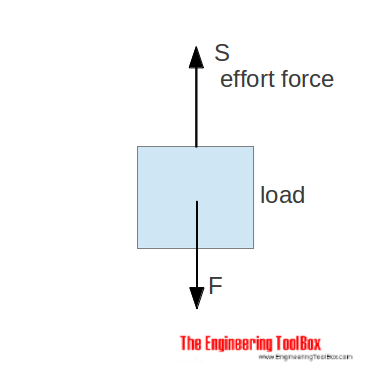Engineering ToolBox - Resources, Tools and Basic Information for Engineering and Design of Technical Applications!

# Movement Ratio

## Machines and movement ratio (velocity ratio).

A machine is a device that can change the magnitude and line of action of a force.Examples are

• pulley systems
• gear systems
• screw-jacks

A simple machine amplifies the input force (effort) to a larger output force (load).

### Movement Ratio

The movement ratio (or velocity ratio) of a machine is defined as the ratio of the distance moved by the effort to the distance moved by the load. Movement ratio can be expressed as

Mr = se / sl

= velocity ratio                         (1)

where

Mr = movement ratio

se = distance moved by the effort   (m, ft)

sl = distance moved by the load     (m, ft)

### Machine Efficiency

The efficiency of a simple machine is defined as the ratio of the force ratio to the movement ratio and can be expressed as

μ = Fr / Mr                       (2)

where

μ = machine efficiency

Fr = force ratio

## Related Topics

• Dynamics - Motion - velocity and acceleration, forces and torque.
• Mechanics - Forces, acceleration, displacement, vectors, motion, momentum, energy of objects and more.

## Engineering ToolBox - SketchUp Extension - Online 3D modeling!

Add standard and customized parametric components - like flange beams, lumbers, piping, stairs and more - to your Sketchup model with the Engineering ToolBox - SketchUp Extension - enabled for use with the amazing, fun and free SketchUp Make and SketchUp Pro .Add the Engineering ToolBox extension to your SketchUp from the SketchUp Pro Sketchup Extension Warehouse!

Translate

## Privacy

We don't collect information from our users. Only emails and answers are saved in our archive. Cookies are only used in the browser to improve user experience.

Some of our calculators and applications let you save application data to your local computer. These applications will - due to browser restrictions - send data between your browser and our server. We don't save this data.

## Citation

• Engineering ToolBox, (2008). Movement Ratio. [online] Available at: https://www.engineeringtoolbox.com/movement-ratio-d_1295.html [Accessed Day Mo. Year].

Modify access date.

. .

#### Scientific Online Calculator3 30